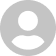1.1

2.81.1
29 March 2003

Word processor for mathematical expressions.

## Overview

CalcPad X is a "word processor" and evaluator for mathematical expressions! CalcPad X lets you type an expression and evaluate it with a single click. Use it as a normal calculator or for evaluating more complex formulas and expressions.

CalcPad X allows you to type in a mathematical expression, edit it for accuracy, format it for easy readability, then evaluate it with single mouse click or key press. And, because it takes full account of standard use of brackets (braces) and mathematical hierarchies (i.e. the orders of precedence of operators - multiplication before addition etc), you can type the expression exactly as it's written. True "wysiwyg" calculating! Each "Pad" (mathematical expression) can be saved for later use (e.g. for a commonly used conversion or formula). Use variables to easily evaluate the same expression with multiple values. Rename variables for clarity and readability. Many scientific and trigonometric functions are included and more will be available with future releases (requests accepted!). CalcPad X also solves quadratic equations and simultaneous linear equations.

## What's new in CalcPad X

Version 1.1 fixes the following:
• Major new feature: Added the ability to solve simultaneous linear equations (maximum of 5 variables).
• Changed the user interface for solving quadratic equations: instead of using a sheet, quadratic equations are now solved in a custom version of the same CalcPad X window, which resizes automatically.
• Added a pop-up button to select the type of evaluation to perform: "General Expression" (the previous default), "Quadratic Equation" and "Simultaneous Equations". The CalcPad X window automatically changes and resizes to accommodate the new kind of evaluation. All three types of expressions can be used simultaneously and all are saved with the window.
• Removed the "Selection" indicator from the "General Expression" window.
• Added quadratic and simultaneous equation help items to the Help menu as an interim measure until the manual can be updated.
• Changed the "Align paper tape" widget to a round button.
• Added a brushed metal version for those who are not yet sick of it!
• Replaced shareware registration screen with a more simple version.
• Updated the FAQ file.
• Added "Brushed Metal" version for those who like the look.
• Fixed a bug that caused CalcPad X to crash if an evaluation was performed with nothing typed in the Pad window.
• Fixed a bug where, in some instances, exponents (e.g. 1e2) were not being evaluated properly.

#### Join over 500,000 subscribers.

Subscribe for our newsletter with best Mac offers from MacUpdate.

Rate this app:31 December 2002